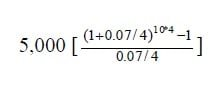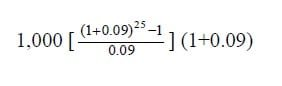Select Page

# Future Value of Annuity Problems and Solutions

## Problem 1: Future value of annuity

What is the future value (as of 10 years from now) of an annuity that makes 10 annual payments of Rs. 5,000, if the interest rate is 7% per year compounded quarterly?

### Solution:Answer: Rs. 286,170.67

Problem 2: Future value of an annuity

Suppose that you start a savings plan by depositing Rs. 1,000 at the beginning of every year into an account that offers 8% per year. If you make the first deposit today, and then three additional ones, how much will have accumulated after four years?

## Problem 3: Future value of annuity due

An annuity makes 25 annual payments of Rs. 1,000 with the first payment coming today. What is the future value of this as of 25 years from now if the interest rate is 9%?

##Problem 4: Future value of annuity table

If at the end of each year a deposit of Rs. 500 is made in an account that pays 8% compounded half yearly, what will the final amount be after five years by factor formula and table?

### Solution:

500 (FVIFA 8%/2, 5*2)

500 (12.006)

>> Download Future Value of Annuity Table.

Problem 5: Future value of annuity factor formula

Your client is 40 years old and wants to begin saving for retirement. You advise the client to put Rs. 5,000 a year into the stock market.  You estimate that the market’s return will be on average of 12% a year. Assume the investment will be made at the end of the year.  How much money will she have by age 65 by factor formula and table?

### Solution:

FVAn = 5,000 (FVIFA 12%, 25)

FVA25 = 5,000 (133.334)

## Problem 6: Future value of growing annuity

Ahmed deposits Rs. 300 at the end of each quarter in his savings account.  If the account earns 5.75% yearly, how much money will he has in 4 years?

## Problem 7: Future value of annuity due and ordinary annuity

If you put Rs. 100 in the market at the end of every year for 20 years at 10%, how much would you end up with? What if you put the Rs. 100 in at the beginning of every year?

## Problem 8: Calculate future value of annuity

You have just finished school and started working full time, so you begin to invest Rs.100 every month in securities. If your securities have an interest rate of 6% compounded semi-annually, how much will you investment be worth in 3 years. Solve by factor formula and table?

### Solution:

100 (FVIFA 6%/2, 36)

100 (63.2760)

## Problem 9: Future value of an annuity table

What is the future value of a five year ordinary annuity, if the annual interest is 10%, and the annual payment is Rs. 50,000; calculate by factor formula and table?

### Solution:

50,000 (FVIFA 10 %, 5)

50,000 (6.1051)

## Problem 10: Future value of an ordinary annuity

You decide to work for next 20 years before an early-retirement.  For your post-retirement days, you plan to make a monthly deposit of Rs. 1,000 into a retirement account that pays 12% p.a. compounded monthly.  You will make the first deposit one month from today. What will be your account balance at the end of 20 years?

## Related Topics

Formula Sheet

Future Value and Present Value Tables

## Related Courses

Principles of Accounting

Cost Accounting

Principles of Finance